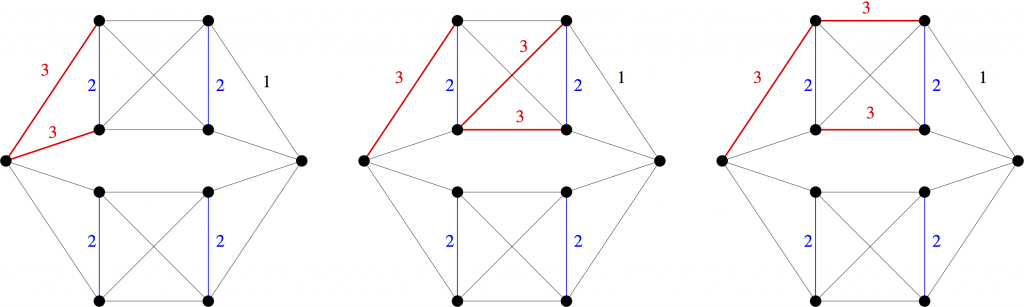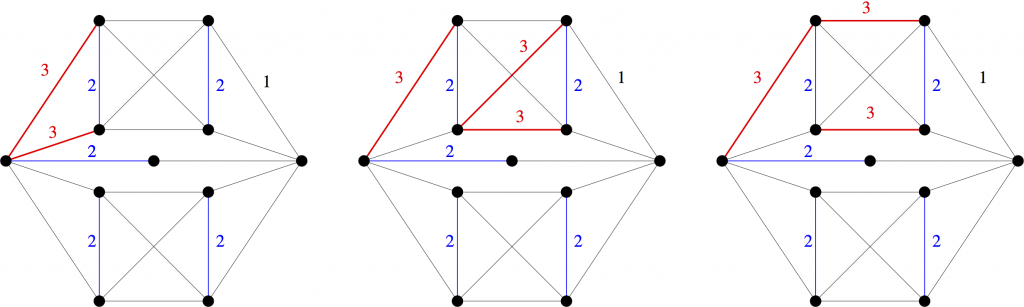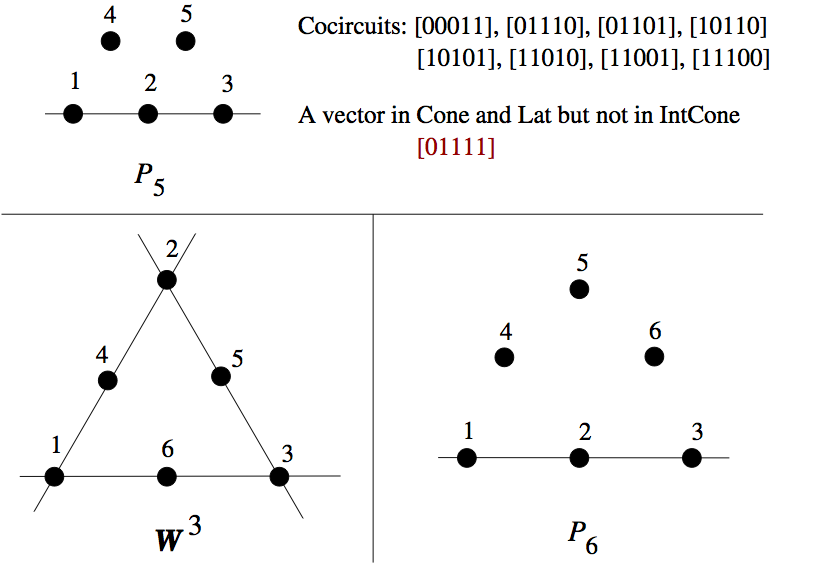# Hilbert Bases of Cuts and Cocircuits

Let $X$ be a set of vectors in $\mathbb{R}^d$.  Three important objects related to $X$ are the cone, lattice, and integer cone generated by $X$.  These are (respectively), the set of all real non-negative combinations, the set of all integer combinations, and the set of all non-negative integer combinations of vectors in $X$.  Clearly, the integer cone is contained in the intersection of the cone and the lattice.  If the integer cone is equal to the intersection of the cone and the lattice, we say that $X$ is a Hilbert basis.

Hilbert bases were introduced by Giles and Pulleyblank  as a tool to study total dual integrality. They are often nicer to work with than arbitrary sets of vectors.  For example, from the computational perspective, membership testing can be much easier for the cone and lattice than for the integer cone.

Given a graph $G$ (or matroid), we may regard subsets of $E(G)$ as characteristic $(0,1)$-vectors. In this way, we can ask whether a family $\mathcal{S}$ of subgraphs of $G$ is a Hilbert basis. In the case that $\mathcal{S}$ is the set of circuits of $G$, there is the following nice theorem of Alspach, Goddyn and Zhang .

Theorem 1 [Alspach, Goddyn, Zhang].  The set of circuits of a graph $G$ is a Hilbert basis if and only if $G$ does not contain the Peterson graph as a minor.

From the matroidal perspective, the difference between circuits and cuts is superficial, so it is very natural to ask the following question.

Question 2.  For which graphs $G$ is the set of cuts of $G$ a Hilbert basis?

Let us define a graph to be cut- (respectively circuit-) Hilbert if its set of cuts (respectively circuits) is a Hilbert basis.  Let $K_{5}^{\perp}$ be the unique 3-connected uncontraction of $K_5$.

Laurent  had previously shown that $K_{5}^{\perp}$ is cut-Hilbert.

In a recent paper of Deshpande, Goddyn and myself , we prove that all graphs without a $K_{5}^{\perp}$-minor are cut-Hilbert.

Theorem 3 [Deshpande, Goddyn, H].  Every graph without a $K_{5}^{\perp}$-minor is cut-Hilbert.

However, somewhat curiously, we also prove that the class of cut-Hilbert graphs is not as well-behaved as the class of circuit-Hilbert graphs.

Theorem 4 [Deshpande, Goddyn, H].  The class of cut-Hilbert graphs is not closed under edge-deletion, edge-subdivision, nor 2-sums.

Note that Theorem 4 is in stark contrast to Theorem 1, where there is an exact excluded-minor characterization for the class of circuit-Hilbert graphs.

I will now give the examples that establish Theorem 4.

It will be important to clarify what we mean by a 2-sum.  A 2-sum of two graphs is obtained by gluing them together along a common edge, and then deleting that edge. A 2-clique-sum of two graphs is obtained by gluing them together along a common edge, but keeping that edge. In contrast to 2-sums, Laurent  also proved that the family of cut-Hilbert graphs is closed under 2-clique-sums.Three different weightings of a non-cut-Hilbert graph $H_1$. Black edges all have weight 1.

Consider the three edge-weightings of the above graph $H_1$.  For a simple graph $G$, it is easy to show that a vector $x \in \mathbb{Z}^{E(G)}$ is in the lattice of cuts of $G$ if and only if $x(C)$ is even for every circuit $C$ of $G$.  One easily checks that this condition holds for each of the above three edge-weightings.  Furthermore, one can also write (but for the benefit of the reader we will not) each of these vectors as real non-negative combinations of cuts of $H_1$. On the other hand, none of these vectors is in the integer cone of cuts of $H_1$ (this is just a (large) finite case check).  This last claim was verified by our silent robot partner Normaliz , but can also be checked by humans (as we do in the paper). Therefore, $H_1$ is not cut-Hilbert.Three different weightings of a non-cut-Hilbert graph $H_2$. Black edges all have weight 1.

Similarly, each of the three edge-weightings of the above graph $H_2$ show that $H_2$ is not cut-Hilbert. Now let $H_3$ be the 2-clique-sum of two copies of $K_{5}^{\perp}$ along the distinguished edge $e$. Since $H_3$ is a 2-clique-sum of cut-Hilbert graphs, $H_3$ is also cut-Hilbert. However, note that $H_1$ is obtained from $H_3$ by deleting an edge and $H_2$ is obtained from $H_3$ by subdividing an edge. This establishes Theorem 4, as promised.

I will finish by discussing the matroid analogue of Question 2.

Question 5.  For which matroids $M$ is the set of cocircuits of $M$ a Hilbert basis?

Luis Goddyn and Marko Mitrovic (an NSERC undergraduate research student of Luis in 2013) made some interesting progress which I will briefly report here. Using Normaliz, the matroid Sage package, and the catalogue of all matroids up to 9 elements, they were able to determine all the non-cocircuit-Hilbert matroids up to 8 elements.  It turns out that there are lots of them.  In particular, of the 2198 matroids with up to 8 elements, 1305 are non-cocircuit-Hilbert.  Below is an image of the three smallest non-cocircuit-Hilbert matroids.If one restricts to binary matroids, then the situation is more manageable. There are only two binary non-cocircuit-Hilbert matroids up to 8 elements, and interestingly, both of them are coextensions of the Fano matroid.  Therefore, the following problem may be doable.

Problem 6.  Characterize the binary matroids whose set of cocircuits is a Hilbert basis.

Note that Problem 6 is open even for graphs.  By Theorem 4, the set of cut-Hilbert graphs is not closed under taking minors, so it does not have an excluded-minor characterization. However, perhaps there is an alternate nice characterization.

References

 F. R. Giles and W. R. Pulleyblank.  Total dual integrality and integer polyhedra.  Linear Algebra Appl., 25:191-196, 1979.

 Brian Alspach, Luis Goddyn, and Cun Quan Zhang.  Graphs with the circuit cover property. Trans. Amer. Math. Soc., 344(1):131-154, 1994.

 Monique Laurent.  Hilbert bases of cuts.  Discrete Math., 150(1-3):257-279, 1996.

 Tanmay Deshpande, Luis Goddyn, and Tony Huynh.  On Hilbert bases of cuts. arXiv.

 Winfred Bruns and Bogdan Ichim.  Normaliz: algorithms for affine monoids and rational cones.  J. Algebra, 324(5):1098-1113, 2010.

## 2 thoughts on “Hilbert Bases of Cuts and Cocircuits”

1. Hi Tony, do we know anything about basis-Hilbert matroids (or spanning-tree-Hilbert graphs)?

Cheers,

Dillon

2. Hi Dillon. Sorry for the late response. Actually, for every matroid M, the set of incidences vectors of bases is always a Hilbert basis. This follows from the Matroid Partition Theorem. In fact, if M has rank r and n elements, then every vector in the integer cone of incidence vectors of bases can be written as a non-negative combination of at most n+r-1 bases.

This site uses Akismet to reduce spam. Learn how your comment data is processed.